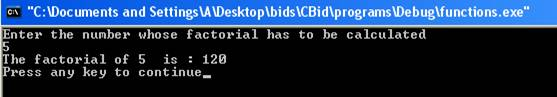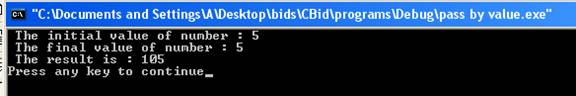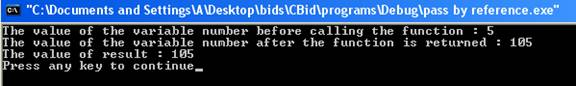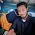## 9.3.14

### Functions in C++

Functions

Before reading this tutorial you should have knowledge of pointers.

Functions are building blocks of the programs. They make the programs more modular and easy to read and manage. All C++ programs must contain the function main( ). The execution of the program starts from the function main( ). A C++ program can contain any number of functions according to the needs. The general form of the function is: -

return_type  function_name(parameter list)
{
body of the function

}

The function of consists of two parts function header and function body. The function header is:-

return_type   function_name(parameter list)

The return_type specifies the type of the data the function returns. The return_type can be void which means function does not return any data type. The function_name is the name of the function. The name of the function should begin with the alphabet or underscore. The parameter list consists of variables separated with comma along with their data types. The parameter list could be empty which means the function do not contain any parameters. The parameter list should contain both data type and name of the variable. For example,

int factorial(int n, float j)

is the function header of the function factorial. The return type is of integer which means function should return data of type integer. The parameter list contains two variables n and j of type integer and float respectively. The body of the function performs the computations.

Function Declaration

A function declaration is made by declaring the return type of the function, name of the function and the data types of the parameters of the function.  A function declaration is same as the declaration of the variable. The function declaration is always terminated by the semicolon. A call to the function cannot be made unless it is declared. The general form of the declaration is:-

return_type function_name(parameter list);

For example function declaration can be
int factorial(int n1,float j1);

The variables name need not be same as the variables of parameter list of the function. Another method can be

int factorial(int , float);

The variables in the function declaration can be optional but data types are necessary.

Function Arguments

The information is transferred to the function by the means of arguments when a call to a function is made.  Arguments contain the actual value which is to be passed to the function when it is called.  The sequence of the arguments in the call of the function should be same as the sequence of the parameters in the parameter list of the declaration of the function. The data types of the arguments should correspond with the data types of the parameters. When a function call is made arguments replace the parameters of the function.

The Return Statement and Return values

A return statement is used to exit from the function where it is. It returns the execution of the program to the point where the function call was made. It returns a value to the calling code. The general form of the return statement is:-

return expression;

The expression evaluates to a value which has type same as the return type specified in the function declaration. For example the statement,

return(n);

is the return statement of the factorial function. The type of variable n should be integer as specified in the declaration of the factorial function. If a function has return type as void then return statement does not contain any expression. It is written as:-

return;

The function with return type as void can ignore the return statement. The closing braces at the end indicate the exit of the function. Here is a program which illustrates the working of functions.

#include<iostream>
using namespace std;
int factorial(int n);

int main ()
{
int n1,fact;
cout <<"Enter the number whose factorial has to be calculated" <<  endl;
cin >> n1;
fact=factorial(n1);
cout << "The factorial of " << n1 << "  is : " << fact << endl;
return(0);

}
int factorial(int n)
{
int i=0,fact=1;
if(n<=1)
{
return(1);
}
else
{
for(i=1;i<=n;i++)
{
fact=fact*i;
}
return(fact);
}
}

The result of the program is:-The function factorial calculates the factorial of the number entered by the user. If the number is less than or equal to 1 then function returns 1 else it returns the factorial of the number. The statement

int factorial(int n);

is a declaration of the function. The return type is of integer. The parameter list consists of one data type which is integer. The statement

cout <<"Enter the number whose factorial has to be calculated" <<  endl;
cin >> n1;
makes the user enter the number whose factorial is to be calculated. The variable n1 stores the number entered by the user. The user has entered number 5. The statement

fact=factorial(n1);

makes a call to the function. The variable n1 is now argument to the function factorial. The argument is mapped to the parameters in the parameter list of the function. The function header is

int factorial(int n)

The body of the function contains two return statements. If the value entered by the user is less than and equal to 1 then value 1 is returned else computed factorial is returned. The type of the expression returned is integer.

Parameter passing mechanism

There are two parameter passing mechanisms for passing arguments to functions such as pass by value and pass by reference.

Pass by value

In pass be value mechanism copies of the arguments are created and which are stored in the temporary locations of the memory. The parameters are mapped to the copies of the arguments created. The changes made to the parameter do not affect the arguments. Pass by value mechanism provides security to the calling program. Here is a program which illustrates the working of pass by value mechanism.

#include<iostream>
using namespace std;
int add(int n);

int main()
{
int number,result;
number=5;
cout << " The initial value of number : " << number << endl;
result=add(number);
cout << " The final value of number : " << number << endl;
cout << " The result is : " << result << endl;
return(0);
}

int add(int number)
{
number=number+100;
return(number);
}

The result of the program is:-The value of the variable number before calling the function is 5. The function call is made and function adds 100 to the parameter number. When the function is returned the result contains the added value. The final value of the number remains same as 5. This shows that operation on parameter does not produce effect on arguments.

Pass by reference

Pass by reference is the second way of passing parameters to the function. The address of the argument is copied into the parameter. The changes made to the parameter affect the arguments.  The address of the argument is passed to the function and function modifies the values of the arguments in the calling function. Here is a program which illustrates the working of pass by reference mechanism.

#include<iostream>
using namespace std;
int add(int &number);

int main ()
{
int number;
int result;
number=5;
cout << "The value of the variable number before calling the function : " << number << endl;
result=add(&number);
cout << "The value of the variable number after the function is returned : " << number << endl;
cout << "The value of result : " << result << endl;
return(0);
}

int add(int &p)
{
*p=*p+100;
return(*p);
}

The result of the program is:-The address of the variable is passed to the function. The variable p points to the memory address of the variable number. The value is incremented by 100. It changes the actual contents of the variable number. The value of variable number before calling the function is 100 and after the function is returned the value of variable number is changed to 105.

After an introduction of functions, let us move on to discuss structures.

#### 2 comments:

1./Program using Constructor
#include
using namespace std;
class checkpelindrom
{
int num;
public:
checkpelindrom();
~checkpelindrom();
void view();
};
checkpelindrom :: checkpelindrom()
{
cout<<"Enter the number\n";
cin>>num;
}
checkpelindrom :: ~checkpelindrom()
{
//cout<<"\n\nDestruction";
}
void checkpelindrom :: view()
{
int a ,temp ,rev=0;
temp=num;
while(temp!=0)
{
a=temp%10;
rev=rev*10+a;
temp /=10;
}
if(rev==num)
cout<<"\nIt is a pelindrom number\n\n";
else
cout<<"\nIt is not a pelindrom number\n\n";

}
int main()
{
checkpelindrom cp;
cp.view();
return 0;
}

2.Abstract Class Example:
Consider the following example where parent class provides an interface to the base class to implement a function called getArea():

#include

using namespace std;

// Base class
class Shape
{
public:
// pure virtual function providing interface framework.
virtual int getArea() = 0;
void setWidth(int w)
{
width = w;
}
void setHeight(int h)
{
height = h;
}
protected:
int width;
int height;
};

// Derived classes
class Rectangle: public Shape
{
public:
int getArea()
{
return (width * height);
}
};
class Triangle: public Shape
{
public:
int getArea()
{
return (width * height)/2;
}
};

int main(void)
{
Rectangle Rect;
Triangle Tri;

Rect.setWidth(5);
Rect.setHeight(7);
// Print the area of the object.
cout << "Total Rectangle area: " << Rect.getArea() << endl;

Tri.setWidth(5);
Tri.setHeight(7);
// Print the area of the object.
cout << "Total Triangle area: " << Tri.getArea() << endl;

return 0;
}

When the above code is compiled and executed, it produces following result:

Total Rectangle area: 35
Total Triangle area: 17

Comment Here